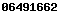## Spring 2017: Data Science and Predictive Analytics (UMich HS650)

Mathematical Foundations | Applied Inference | Algorithms and Programming

This is a voluntary self-assessment that may help you determine if you have all the necessary background to successfully complete the MOOC in due time, or if some remediate work may be helpful to you before you start this course. Completing any preliminary training is not absolutely required but may be advisory in certain situations. This Pre-Test is provided as a self-assessment, and is not evaluated as part of the course, nor does it factor into the assessment of your work in the course or your course grade.

### Mathematical Foundations

• #### Calculus of differentiation and integration

• What do the solutions of the following equations represent?
• -y<5+3x
• -2x +3y -7z = 7
• $$\begin{array}{lcl} x+2y-z &=& 1 \\ 2x-2y+4z &=& -2 \\ -x+0.5y-z &=& 0 \end{array}$$
• $$\int_{-\infty}^{\infty}{e^{-\frac{x^2}{2}} dx} =y$$
• What are the local and global extrema of the function $$f(x)=x^3 + 3x^2 − 2x + 1$$ over the interval $$[−4, 2]$$?
• #### Numerical methods

• What does this series represent $$\sum_{n=0}^{\infty} { \frac{(-1)^n}{(2n+1)!} x^{2n+1} }?$$
• Starting with $$x_o=3$$, complete 3 iterations of the Newton-Raphson method, $$x_{n+1}=x_n-\frac{f(x_n)}{f'(x_n)}$$, to estimate a root of $$f(x)=x^3-20$$, i.e., find a solution to this equation: $$f(x)=x^3-20=0$$.
• #### Matrix manipulations:

If $$A_{3\times 3}= \begin{pmatrix} 3 & 2 & -1 \\ 2 & -2 & 4 \\ -1 & 1/2 & -1 \end{pmatrix}$$ and $$x_{3\times 1}= \begin{pmatrix} 1 \\ -2 \\ -2 \end{pmatrix}$$, what is the product $$A_{3\times 3} \times x_{3\times 1}$$?

### Applied Inference

• #### Data modeling

• Give examples of linear, polynomial and exponential models of an outcome ($$Y$$) in terms of some observed input ($$X$$).
• What is a graph?
• #### Probabilities

• Suppose we draw 2 balls randomly, one at a time without replacement, from an urn containing 4 black and 3 white balls, otherwise identical. What is the probability that the second ball is black?
• Suppose a patient visits a primary care clinic and is seen by a male provider not wearing a badge or other insignia. Assume that the number of primary care physicians in the US is 435,000, the number of practicing nurses is 4,500,000, and the reported gender distributions in the 2 professions (F:M ratios are 1:2 for physicians and 12:1 for nurses). Using only this information, to address the clinician appropriately, the patient is trying to figure out if he is more likely to be a doctor or a nurse (assuming these are the only options in this clinical setting). Compute and interpret the odds likelihood ratio of the clinician being a nurse.
• #### Formulation of Research Questions (Hypotheses), EDAs, and Confirmatory Data Analyses

• What is a statistic?
• What is a p-value?
• How to interpret a confidence interval of a parameter estimate?

Examples of MOOC courses that provide some foundations in Applied Inference include:

### Algorithms, Programming, Computational Background

• #### Sorting Algorithms, and complexity

• Explain why the computational complexity of the Bubble Sort algorithm is $O(n^2)$. This algorithm works by comparing each number in the list with the item next to it, and swaps them if needed to reorder the data from the smallest to the largest element.
• List 2 examples of programming syntax for sorting an array in different languages; e.g., in Java: int[] array_raw = {2,3,4,1,5,2,6}; array_sorted = Arrays.sort(array_raw);
• #### OOP, objects

• What is inheritance in object oriented programming?
• Write a simple OOP program (use any language) representing a Shape class:
• Define a Shape object as a 2D polygon that has: a name, a perimeter, and an area.
• Define a Circle, as a Shape, inheriting the characteristics of Shape.
• Define a Triangle, including a special indicator for equilateral, isosceles or scalene (no 2 sides are the same) triangles.
• Overwrite the “area” method for isosceles triangles.
• #### Data Formats, APIs

• What are binary, ASCII, structured, and unstructured data?
• What is JSON?
• Explain this GoogleMaps API call (you can save this in index.html file and load it in your browser to see the result):
<!DOCTYPE html> <html> <body> <h1>Google Map API</h1> <div id="map" style="width: 100%; height: 500px"></div> <script type="text/javascript"> function myMap() { var mapCanvas = document.getElementById("map"); var mapOptions = { center : new google.maps.LatLng(42.284199, -83.738072), zoom : 15 }
var map = new google.maps.Map(mapCanvas, mapOptions); } </script> <script src="https://maps.googleapis.com/maps/api/js?callback=myMap" type="text/javascript"></script> </body> </html>

Examples of MOOC courses that provide some foundations in Algorithms, Programming, and Computational background include:

SOCR Resource Visitor number